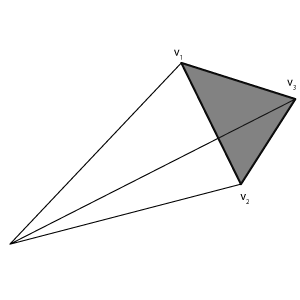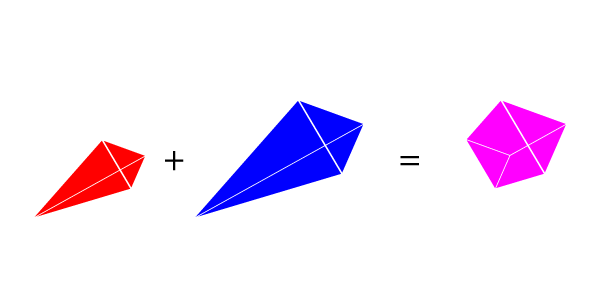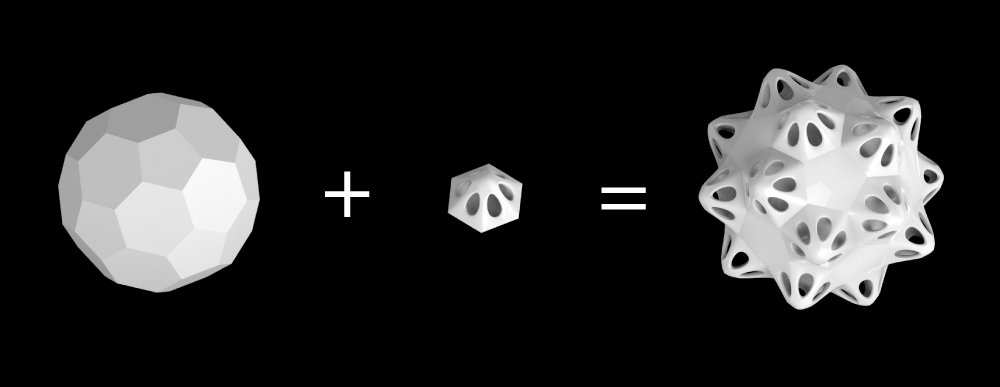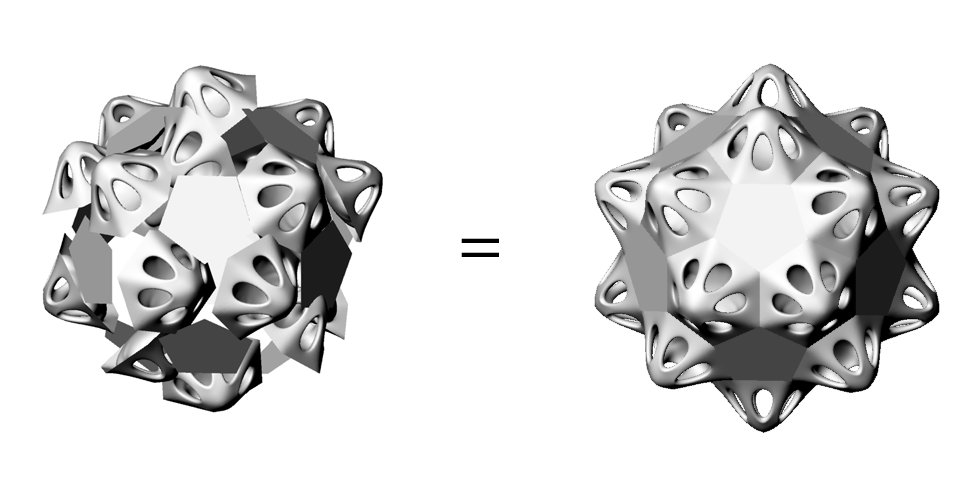# Calculating the volume of a mesh

In order to generate the price of a custom design on the fly, we need to calculate the volume of the piece for 3d printing. By constantly updating the volume, the customer gets instant feedback on how their changes are affecting price. Calculating the volume of a mesh is a relatively simple and well-known problem, and I’ll go over the straight forward case as well as an optimization we’ve incorporated into our latest project.

The Basics

The idea behind calculating the volume of a mesh is to calculate a volume for each triangle of the mesh and add them up. Now, a triangle itself does not have volume; it is two dimensional. Instead we calculate the volume of a tetrahedron which goes from the origin (0,0,0) to the triangle.There is neat equation for calculating volume of a tetrahedron. Given a triangle’s points v1, v2, v3 the tetrahedrons volume is$V = \frac16 (v_1 \times v_2) \cdot v_3$

Another way to express this is if we have a 3×3 matrix, where each row is one of our vertices the volume is a sixth of the determinant. The division by six comes from the fact that determinant is actually the volume of the parallelpiped formed by the three vectors, and you can stuff 6 tetrahedrons into the parallelpiped.But wait, if I add up all these tetrahedrons don’t I get a mess of overlapping volumes that go to the origin? Yes, but the key thing is that these volumes are signed, so they can be negative depending on the vertex winding. If my points go one way (v1->v2->v3) I get a positive volume and if they go the other way (v1->v3->v2) I get a negative volume. Faces pointing out add to the total volume and faces pointing in subtract. What is left is only the volume inside my object. To get the total volume of a mesh, we go through each triangle, compute its signed volume, and add them up.

Below is some javascript code for computing the volume of a mesh stored in something like a Vertex Buffer Object.

Volume of repeated elements

Now, onto the good stuff. What happens if you have an object that is made of (at least in part) an aggregate of a bunch of identical but complex parts. I don’t mean a booleaning together primitives, but you could imagine something like a buckyball where each face is articulated with some kind of intricate shape. The brute force approach would be to move and rotate the shape to the proper position then go through each triangle and calculate the volume. This means you have to calculate a transform on each of the points of your shape, and then go through each triangle. If your shape has 1000 triangles and you have 100 shapes, that ends up being a lot calculation. We can drastically increase the efficiency of this by computing a “general volume” for the shape once, and applying our transforms only to that simplified representation. But what does this general volume look like?Rotation

The key idea behind this general volume is the fact that volume is rotation invariant. This is one of the basic results of differential geometry. It is intuitively obvious; no matter how I orient an object in space its volume does not change. What is less intuitive is that the same thing holds true for the signed volume of open shapes. Mathematically this can be seen easily by noting that the volume is the determinant of a matrix, and the rotation matrix has a determinant of 1. The determinant of one matrix multiplied by another is the multiplication of their individual determinants. So, I can rotate my primitive element however I want, and the volume stays the same. If I was only rotating my shape, then I could calculate the volume of my shape once and multiply it by the number of shapes I have.

TranslationThat only leaves translation or moving my shape around in space. We can look at this intuitively in a simplified 2D example with the area of a single line segment. The area of a triangle is one half base time height. If I translate my line segment in the x direction by some amount, I am adding that amount to my height. So the area of my translated line is:$A_t = \frac12 b (h+x) = A + \frac12 bx$

I take my original area, and I add on some amount multiplied by my x translation. Though this is a very dumbed down example, we can do essentially the same thing for each axis (x,y,z) in our volume.

Nitty gritty

To see how this idea applies to our volume calculation, we can look at the expanded equation for the volume of each triangle, where$v_1 = (x_1,y_1,z_1)$$V = x_1y_2z_3-x_2y_1z_3+x_3y_1z_2-x_1y_3z_2+x_2y_3z_1-x_3y_2z_1$

That may look like a lot of equation, but if look at each individual term, we notice that it is the sum of terms that look like an x component times a y component times a z component. Isolating an arbitrary term if we translate along one axis, we get something similar to our simple 2d example:$V_t = x_1y_2(z_3+z_t) = V + x_1y_2z_t$

You might say, that is only translating one direction, what happens when you are doing an arbitrary translation? It turns out because of the way the terms are organized in positive and negative pairs, every term besides the one in one directional example cancels out. So our general volume becomes a vector which sums up the terms for each axis. Each axis term is all the pairs of vertex coordinates that don’t include that axis:$V_x = y_2z_3-y_1z_3+y_1z_2-y_3z_2+y_3z_1-y_2z_1$$V_y = x_1z_3-x_2z_3+x_3z_2-x_1z_2+x_2z_1-x_3z_1$$V_z = x_1y_2-x_2y_1+x_3y_1-x_1y_3+x_2y_3-x_3y_2$

Just like our regular volume, we can just add these up for each triangle in our shape. Our final volume calculation becomes:$V_t(x_t,y_t,z_t) = V+V_xx_t+V_yy_t+V_zz_t$

This is great not only because it allows us to calculate the volume without going through each triangle, but also we don’t even have to know how our shape is oriented! This leads to some strange facts, like if I randomly rotate each of my shapes but put them in the same spot it has the same volume.### MoMA Acquires First Kinematics Dress

#### One Comment

1.Testing